Next: JFET Common Drain Amplifier Up: Transistor Circuits Previous: Small-Signal AC Model

# JFET Common Source Amplifier

The common source configuration for a FET is similar to the common emitter bipolar transistor configuration, and is shown in figure 5.17. The common source amplifier can provide both a voltage and current gain. Since the input resistance looking into the gate is extremely large the current gain available from the FET amplifier can be quite large, but the voltage gain is generally inferior to that available from a bipolar device. Thus FET amplifiers are most useful with high output-impedance signal sources where a large current gain is the primary requirement. The source by-pass capacitor provides a low impedance path to ground for high frequency components of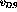and hence AC signals will not cause a swing in the bias voltage.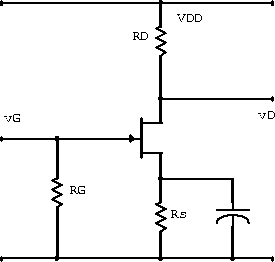Figure 5.17:  JFET common source amplifier.

Since the FET gate current is small we can make the approximations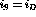and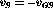: the source is positive with respect to the gate for reverse-bias. Since at low frequencies we can ignore the capacitor the source voltage is given by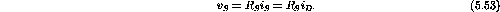Using the transconductance equation we can write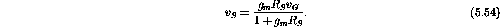With the approximationwe can write the drain voltage as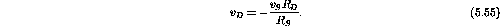The voltage gain is thus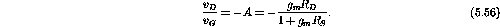If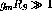, this reduces to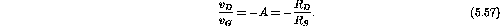Doug Gingrich
Tue Jul 13 16:55:15 EDT 1999